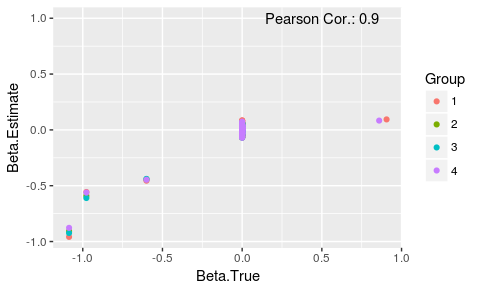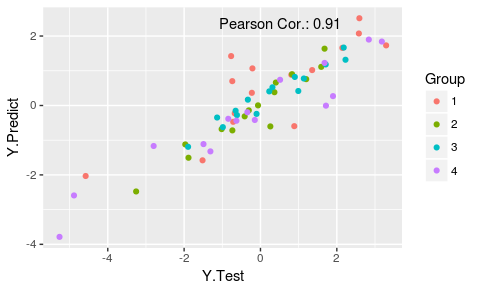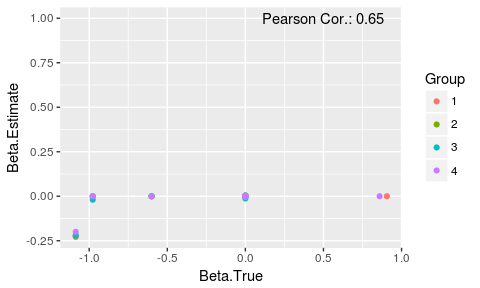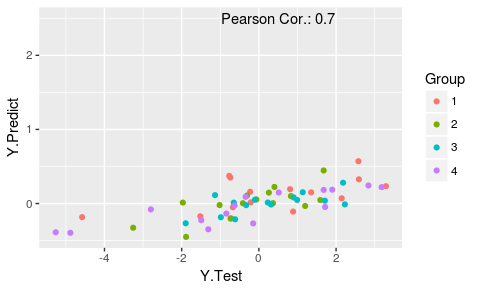# Using the fuser package for prediction over subgroups

#### 2018-06-17

The fuser package implements l1 penalised regression with fusion over subgroups, as described in Dondelinger (2016). The fusion penalties allow for information sharing across regression parameters for different subgroups in heterogeneous datasets. Currently, the functionality of the package is limited to estimating the linear coefficients, although we intend to extend this with functions for prediction, cross-validation and visualization.

The package implements l1 and l2 fusion penalties; l1 penalization is optimized via a proximal gradient algorithm, while l2 penalization uses a reformulation of the model to apply the glmnet package for estimation.

## Running Example

We will generate example data from a simple model with 4 groups, 100 covariates and 15 samples per group. Roughly half of the non-zero linear coefficients used for generating the data will be shared between all groups. Note also that shared coefficients are negative, independent coefficients are positive.

# Test of using fusion approach to model heterogeneous
# datasets
set.seed(123)

# Generate simple heterogeneous dataset
k = 4 # number of groups
p = 100 # number of covariates
n.group = 15 # number of samples per group
sigma = 0.05 # observation noise sd
groups = rep(1:k, each=n.group) # group indicators

# sparse linear coefficients
beta = matrix(0, p, k)
nonzero.ind = rbinom(p*k, 1, 0.025/k) # Independent coefficients
nonzero.shared = rbinom(p, 1, 0.025) # shared coefficients
beta[which(nonzero.ind==1)] = rnorm(sum(nonzero.ind), 1, 0.25)
beta[which(nonzero.shared==1),] = rnorm(sum(nonzero.shared), -1, 0.25)

X = lapply(1:k, function(k.i) matrix(rnorm(n.group*p),n.group, p)) # covariates
y = sapply(1:k, function(k.i) X[[k.i]] %*% beta[,k.i] + rnorm(n.group, 0, sigma)) # response
X = do.call('rbind', X)

# Generate test dataset
X.test = lapply(1:k, function(k.i) matrix(rnorm(n.group*p),n.group, p)) # covariates
y.test = sapply(1:k, function(k.i) X.test[[k.i]] %*% beta[,k.i] + rnorm(n.group, 0, sigma)) # response

## L1 Fusion

We use the function fusedLassoProximal to estimate the linear coefficients via an l1 fusion penalty.

library(fuser)
library(ggplot2)

# Pairwise Fusion strength hyperparameters (tau(k,k'))
# Same for all pairs in this example
G = matrix(1, k, k)

# Use L1 fusion to estimate betas (with near-optimal sparsity and
# information sharing among groups)
beta.estimate = fusedLassoProximal(X, y, groups, lambda=0.001, tol=9e-5,
gamma=0.001, G, intercept=FALSE,
num.it=2000) 

Alternatively, we can use the C++ implementation of the proximal algorithm by Oliver Wilkinson. This can be faster on some systems, especially for large numbers of subgroups.

beta.estimate = fusedLassoProximal(X, y, groups, lambda=0.001, tol=9e-5,
gamma=0.001, G, intercept=FALSE,
num.it=2000, c.flag=TRUE) 
plotting.frame = data.frame(Beta.True=c(beta),
Beta.Estimate=c(beta.estimate),
Group=factor(rep(1:k, each=p)))

correlation = round(cor(c(beta.estimate), c(beta)), digits=2)

ggplot(plotting.frame, aes(x=Beta.True, y=Beta.Estimate, colour=Group)) +
geom_point() +
annotate('text', x=0.5,y=1, label=paste('Pearson Cor.:', correlation))Then we can use the estimated coefficients to predict the response for unseen data points.

# Predict response based on estimated betas
y.predict = sapply(1:k, function(k.i) X.test[[k.i]] %*% beta.estimate[,k.i]) 
plotting.frame = data.frame(Y.Test=c(y.test),
Y.Predict=c(y.predict),
Group=factor(rep(1:k, each=n.group)))

correlation = round(cor(c(y.test), c(y.predict)), digits=2)

ggplot(plotting.frame, aes(x=Y.Test, y=Y.Predict, colour=Group)) +
geom_point() +
annotate('text', x=0.5,y=2.35, label=paste('Pearson Cor.:', correlation))## L2 Fusion

Estimating the linear coefficients via an l2 fusion penalty is done by reformatting the data in block diagonal form to fit it into the standard lasso format (via function generateBlockDiagonalMatrices) and then invoking the wrapper function fusedL2DescentGLMNet that calls the glmnet package.

# Generate block diagonal matrices for L2 fusion approach
transformed.data = generateBlockDiagonalMatrices(X, y, groups, G)

# Use L2 fusion to estimate betas (with near-optimal information sharing among groups)
beta.estimate = fusedL2DescentGLMNet(transformed.data$X, transformed.data$X.fused,
transformed.data\$Y, groups, lambda=c(0,0.001,0.1,1),
gamma=0.001)

# Returns a beta matrix for each lambda value, so we extract the one we think is optimal.
beta.estimate = beta.estimate[,,2]
plotting.frame = data.frame(Beta.True=c(beta),
Beta.Estimate=c(beta.estimate),
Group=factor(rep(1:k, each=p)))

correlation = round(cor(c(beta.estimate), c(beta)), digits=2)

ggplot(plotting.frame, aes(x=Beta.True, y=Beta.Estimate, colour=Group)) +
geom_point() +
annotate('text', x=0.5,y=1, label=paste('Pearson Cor.:', correlation))Again we can use the estimated coefficients to predict the response for unseen data points.

# Predict response based on estimated betas
y.predict = sapply(1:k, function(k.i) X.test[[k.i]] %*% beta.estimate[,k.i]) 
plotting.frame = data.frame(Y.Test=c(y.test),
Y.Predict=c(y.predict),
Group=factor(rep(1:k, each=n.group)))

correlation = round(cor(c(y.test), c(y.predict)), digits=2)

ggplot(plotting.frame, aes(x=Y.Test, y=Y.Predict, colour=Group)) +
geom_point() +
annotate('text', x=0.5,y=2.5, label=paste('Pearson Cor.:', correlation))Dondelinger, Frank. 2016. “High-Dimensional Regression over Disease Subgroups.” ArXiv. https://arxiv.org/abs/1611.00953.